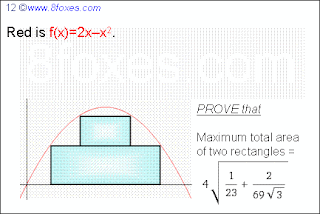## Saturday, March 6, 2010

### Fox 12

Yu sent a confirmed solution.http://www.8foxes.com/

1.Reduce this Problem to half of Problem - x=x+1, g(x)=f(x+1)=1-x^2. We can take two point 0<x1<x2<1.

S(x1,x2)=g(x2)*x2+(g(x1)-g(x2))*x1
Solve system
dS(x1,x2)/dx1=0
dS(x1,x2)/dx2=0
we find x2=sqrt(207+46*sqrt(3))/23 and x1=(sqrt(207+46*sqrt(3)))^3/3174-3*sqrt(207+46*sqrt(3))/46
and 2*S(x1,x2)=4*sqrt(207+46*sqrt(3))/69 is equal to answer on picture problem 12.

2.Thank you Yuv-k for this good solution.